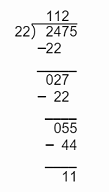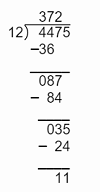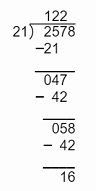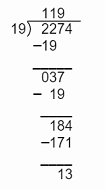### Division - Worksheets

CBSE Worksheet-01

Class - IV Mathematics – Division

Time Allowed: 30 minutes

1. Fill in the blanks with suitable words:
1. Division by zero is ______.
2. Division by _______ is not allowed.
3. In a division sum, the number we divide is called _________.
4. 9321$÷$_____ = 9321
5. If any number other than Zero (0), is divided by itself the quotient will be __________.
2. State whether each of the following statement is True or False:
1. In division, the number by which we divide is called the divisor.
2. In division, the number by which we divide is called dividend.
3. When the divisor is 1 the quotient will be the same number as dividend.
4. [Dividend $×$ Quotient]+ Remainder = Divisor.
5. 123456 $÷$1 = 123456.
3. Gangu also has a bigger box in which he packs 12 laddoos. How many boxes does he need for packing 60 laddoos?
1. 5
2. 4
3. 7
4. 6
4. John earns Rs 3,76,224 per annum, what is his monthly salary?
1. 42,352
2. 31,352
3. 41,352
4. 32,352
5. Solve: 428 $÷$ 2
1. 216
2. 241
3. 217
4. 214
6. Nancy needs 5 lemons to make a glass of orange juice. If Nancy has 250 oranges, how many glasses of orange juice can she make?
1. 100
2. 50
3. 250
4. 60
7. A Fruit seller bought 7300 oranges out of these, 64 were found rotten. Remaining oranges were packed equally in 67 boxes. Find the number of oranges in each box.
1. 106
2. 101
3. 108
4. 118
8.  a) In a division sum, the number we divide is called i) Divisor b) The number by which we divide is called ii) Quotient c) When the divisor is the same number as the dividend the quotient will be iii) Dividend d) The answer to a division problem is called iv) 1
9. If 9975 kg of wheat is packed in 95 bags, how much wheat will each bag contain?

10. What least number should be subtracted from 4320 so that the remainder is exactly divisible by 47?

11. A truck can carry 5000 brick. How many trucks do we need to carry 1,00,000 brick? If the cost of 1 truck of brick is Rs 15000 then find the cost of 1 brick.

12. 6 water tanks of equal capacity hold 468 litres of water.

1. The capacity of each tank?
2. What is the cost of each tank if 6 tanks cost Rs 1260?
3. The number of bottles that can be filled from the tank if 1 bottle contains 3ltr?
13. Solve:

1. 9 pairs of shoes cost Rs 5607. How much does a pair cost?
2. 5 bags contain 4205 kg of rice. How much rice is there in 1 bag?
14. Solve the following:

1. A lady paid Rs 90 for 5 cans of a cold drink. How much did she pay for each cold drink can?
2. A satellite made 128 orbits around the earth in 8 days. How many orbits did it make each day?
3. The product of two numbers is 15,808. One number is 26. Find the other number.
4. A book contains 1024 pages. Ayushree said she would read 16 pages a day. How many days will it take her to read the book?
15. Ritesh has Rs. 3545624 with him. He divided his money in four equal parts. He gave one part for the welfare of forest life programme, one part to tiger conservation program and one part for the conservation of the endangered animals. If Ritesh buys a bicycle of Rs.15800 from the remaining money, then find how much money he gave and how much money was left with Ritesh?

16. Find quotient and remainder in each of the following.

1. 2475 $÷$ 22
2. 4475 $÷$ 12
3. 2578 $÷$ 21
4. 2274 $÷$ 19

CBSE Worksheet-01
Class - IV Mathematics – Division

Solution

1. Fill in the blanks with suitable words:

1. Undefined.

2. 0, because if any number is divided by 0 the result becomes undefined.

3. Dividend.
In division, the number which we divide is called the dividend. The number by which we divide is called the divisor. The result obtained is called the quotient.

4. 1
9321$÷$___ = 9321
___ = $\frac{9321}{9321}$
___ = 1

5. 1
For example: 4$÷$4 = 1

2. State whether each of the following statement is True or False:

1. True, in the division, the number by which we divide is called the divisor.
Eg. when we divide 21 by 3, here 21 is dividend, 3 is divisor.

2. False
In division, the number by which we divide is called the divisor.
Eg. We divide 217 by 4, then 4 is divisor, and 217 is the dividend.

3. True, when the divisor is 1 the quotient will be the same number as dividend.
Eg. when 200 is divided by 1 then quotient is 200.

4. False , [Divisor $×$ Quotient]+ Remainder = Dividend.
Eg. when we divide 25 by 3 we can write, 3 $×$8+1 = 25

5. True
123456 $÷$1= 123456
Here, divisor is 1 hence, quotient is equal to dividend.

3. (a) 5
Explanation: 1 box can pack 12 laddoos.
No. of boxes required to pack 60 laddoos = 60 / 12 = 5
4. (b) 31,352
Explanation:
Annual salary is Rs 376224
So, monthly salary = 376224$÷$12 = Rs 31,352
5. (d) 214
Explanation: 428 / 2 = 214
6. (b) 50
Explanation: 50 is the correct answer.
5 lemons = 1 glass of orange juice
Total = 250 oranges
glasses of orange juice made from 250 oranges = 250$÷$5 = 50
7. (c) 108
Explanation:
Total number of oranges bought = 7300
No. of oranges rotten = 64
No. of oranges left un rotten = 7300 - 64 = 7236
No. of boxes used for packing 7236 oranges = 67
No. of oranges in 1 box = 7236 / 67 = 108
8. (a) - (iii), (b) - (i), (c) - (iv), (d) - (ii)

9. Wheat packed in 95 bags = 9975 kg
then, wheat packed in 1 bag = 9975 $÷$ 95 = 105
So, 105 kg of wheat is packed in each bag.

10. Number = 4320
now after performing division
4320$÷$ 47 = $91\frac{43}{47}$
remainder = 43
now, the remainder is subtracted from 4320
4320 - 43 = 4277
So, 43 is the least number that should be subtracted from 4320

11. Total number of brick = 1,00,000 brick
1 truck carry = 5000 brick
Then, trucks required to carry all brick = 100000$÷$5000 = 20 trucks
Now, the cost of 1 brick = 15000$÷$5000 = Rs 3
So, 20 trucks required to carry 1,00,000 bricks and the cost of 1 brick is Rs 3

12. 6 water tanks of equal capacity hold 468 litres of water.

1. Total water available = 486 litres
Total number of tanks = 6
then, water hold in 1 tank = 486$÷$6 = 81 litres
So, 81 litres is the capacity of one tank.
2. Total cost of 6 water tank = Rs 1260
then, cost of 1 water tank = 1260 $÷$ 6 = 210
So, the cost of 1 water tank = Rs 210
3. Total water available = 486 litres
Capacity of 1 bottle = 3 litres
then, number of water bottle require = 486 $÷$ 3 = 162
So, 162 bottles can be filled.
1. Total cost of 9 pair of shoes = Rs 5607
then, the cost of 1 pair = 5607 $÷$ 9 = Rs 623
So, Rs 623 is the cost of one pair of shoes.
2. Total rice in 5 bags = 4205 kg
then, one bag contain = 4205 $÷$ 5 = 841 kg
So, 841 kg of rice is there in 1 bag.
1. Cost of 5 cold drink cans = Rs 90
then, cost of each cold drink can = 90 $÷$ 5 = Rs 16
2. Orbit made by satellite in 8 days = 128 orbits
then, orbits it make each day = 128 $÷$ 8 = 16
3. The product of two numbers = 15,808
1st number = 26
then, the 2nd number = 15808 $÷$ 26 = 608
4. Total pages in book = 1024 pages
Ayushree read 16 pages a day
then, days need to read the book = 1024 $÷$ 16 = 64 days
13. Total money Ritesh have = 3545624
He divided into four equal part,
then, money he divided equally = 3545624 $÷$ 4 = Rs 886406
He gave three equal part as donation
then, the money he donate = 886406 $×$ 3 = Rs 2659218
now, the money he have = 3545624 - 2659218 = Rs 886406
Out of left money he buys a bicycle of Rs 15800
now, the money left with Ritesh = 886406 - 15800 = Rs 870606

1. $2475÷22$Quotient =112 & Remainder = 11
2. $4475÷12$Quotient =372 & Remainder = 11
3. $2578÷21$Quotient =122 & Remainder = 16
4. $2274÷19$Quotient =119 & Remainder = 13